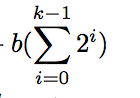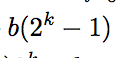Exam prep for COMP 410 Data Structures at UNC, now taught as COMP 210.

```Comp 410 Exam 3

Try to break things down into recursion if it's not a recursive algorithm, realize that things are done in steps and then write the recursive code for completing the action. From there, it should be pretty easy to solve the O(n) equation for the code. You would write it with T(n-1) in the formula if the code calls the n-1 case. So for instance if you had a for loop over n values in the code, called the n-1 case, and also had some other constant value operations, you would have T(n) = T(n-1)+bn+c.

Then you expand the T(n-1) case to be T(n-2) + b(n-1) + c inside this equation and you can continue this for however many steps as you need until you see a pattern emerge that allows you to rewrite the equation without T(N)'s. That way, you should have some sigmas or something and you'll end up getting some polynomial that you can find the O(N) of.

For Binary search, we determine T(1) = a (base case, essentially). And T(n) <= T(n/2) + b. Because we know the recurrence ends up halving n each time, we should come up with an integer k such that 2^(k-1) < n <= 2^k. Then, subbing in and substituting essentially, we have it that:

T(n) <= T(2^k)
T(n) <= T(2^k-1) +b
T(n) <= T(2^k-2) +2b
…
T(n) <= T(1) + kb.

Which is to say that we've done k iterations of b time. So now, we need to solve for k in terms of how we defined it. So we have

2^k-1 < n
(k-1) < log(n)
k<log(n)+1 . We can plug this into our equation for T(n) and blah blah blah we essentially just get O(logN) runtime which is the important part.

————————————————————————————————————————
In instances where we have an iterative program, we can think about it recursively to find the bigOh.

Mainly, we should focus on the act of unwrapping. So given a T(n) <= T(n-1) + bn + c, we can plug in T(n-2)+b(n-1)+c in for T(n-1). Group the n with the n-1, with the n-2, etc. This is the primary concern as this should lead to a summation if one exists.

NOTE: IF YOU HAVE A RECURRENCE THAT GOES T(N) <= T(N/2) + b, then let 2^k-1 < n <= 2^k. Plug in 2^k in for k, then unwrap (you should have k decrementing by one on each step). Once you do this, you should have an expression in terms of constants and k as your variable. Now you need to solve for k in terms of n, so you essentially should just do a log base 2 of each side of the original equality and you’ll get k<log(n) +1 or something.

Remember some basic summations: sum from i to k of 2^i is 2^k+1.

————————————————————————————————————————

Trees

The height node is the longest distance from that node to a leaf. The depth of a node is the distance from that node to the root.

If there is a path from n1 to n2, then n1 is an ancestor of n2 and n2 is a descendant of n1. Proper ancestor and descendant is when they follow that property and they are not the same node.

Because in a tree a node may have many children, it's an inefficient use of memory to have every child stored as a link. So instead, have every node point to "firstChild" and "nextSibling". That way you can traverse however many children there are of a node without the parent storing all of the pointers to its children.

This results in trees that look something like this:

A ———— B
|                  |
C—D          E—F——G——H
|      |
i      J—K

————————————————————————————————————————

Comments regarding test on Piazza:

Writing code that determines whether there exists an integer i such that A[i]=i in an array of integers satisfying A < A < A < ... < A[A.length-1]

To solve this, we would start at the center, and compare the A[mid] value to mid, if A[mid]<mid, then we know that we should need to look higher, otherwise we would look in the bottom half. We continue this recessively until we find the result. This would take O(logN).

NEED TO KNOW FOR THE TEST:

Test involved:

O(n) is similar to <= (grows no faster than a given function. So this is an upper bound.)

Omega(n) is similar to >= (grows at least as fast as a given function. So this is a lower bound. )

Theta(n) is similar to == (grows at the same rate. This is true if both O(n) and Omega(n) is true).

Question in textbook that test question was based on:

A full node in a binary tree is a node with two children. Prove that the number of
full nodes plus 1 is equal to the number of leaves in a non empty binary tree.

Question in test:

Suppose minimum of n nodes, maximum m leaves. n= L + f.
= m + L + m-1
implies n = 2m + L

LAST MINUTE THINGS TO REVIEW:

Theta(n) is the one that’s ==

Remember if T(n/2), set 2^k-1<n<2^kis equal toGroup the n with the n-1, with the n-2, etc. This is the primary concern as this should lead to a summation if one exists.

```